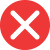calculate

Correct spelling, explanation: the verb calculate comes from the Latin word calculus. Similarly to many words of Latin origin, the pronunciation changed over time, and the word calculus started to function in English as a noun. In order to convert the noun into a verb, the Latin suffix -us was changed to an English verb suffix ate.

Definition of calculate:
verb
1. to count, apply mathematics, like adding, multiplying, etc.
We will calculate the wedding’s attendees by adding all single guests and their plus ones.
The pi number is very useful to calculate the size of circular objects.calulate

Incorrect spelling, explanation: calculus is a Latin word and the base for the verb calculate. Calulate, however, is an incorrect word, as it is not based on anything meaningful. The structure of the word suggests that the base word here is calul or calulus, and these simply don’t exist. And if you struggle with the word calculate, think of the pronunciation and remember to put c where the k sound is present.caluculate

Incorrect spellingcaluclate

Incorrect spellingcallculate

Incorrect spelling